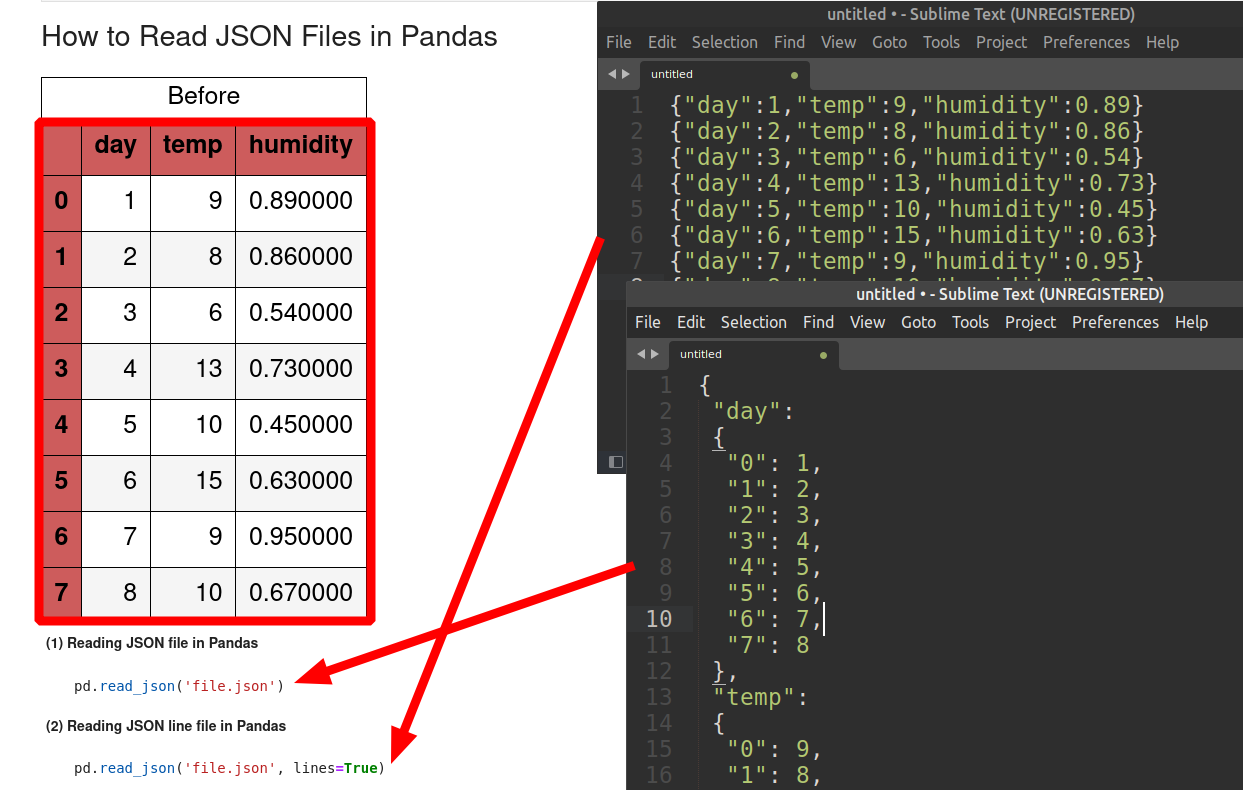In this tutorial, we'll focus on reading JSON files with Pandas and Python. We will cover reading JSON files and JSON lines( read the file as JSON object per line).

(1) Reading JSON file in Pandas

``````pd.read_json('file.json')
``````

(2) Reading JSON line file in Pandas

``````pd.read_json('file.json', lines=True)
``````

## Setup

In our example we'll be using a DataFrame with the next data.

``````import pandas as pd

data = {'day': [1, 2, 3, 4, 5, 6, 7, 8],
'temp': [9, 8, 6, 13, 10, 15, 9, 10],
'humidity': [0.89, 0.86, 0.54, 0.73, 0.45, 0.63, 0.95, 0.67]}

df = pd.DataFrame(data=data)
``````

We will use also a file called 'file.json' which can be exported from this DataFrame by:

``````df.to_json(orient='columns')
``````

then we can read it again to DataFrame with `read_json()`:

``````pd.read_json(df.to_json(orient='columns'), orient='columns')
``````

The content of 'file.json':

``````{
"day":{
"0":1,
"1":2,
"2":3,
"3":4
},
"temp":{
"0":9,
"1":8,
"2":6,
"3":13
},
"humidity":{
"0":0.89,
"1":0.86,
"2":0.54,
"3":0.73
}
}
``````

## 1: Read JSON file with Pandas

To read a JSON file named 'file.json' we can use the method `read_json()`. The official documentation is placed on link: pandas.read_json

By default the method is reading `orient='columns'`:

``````pd.read_json('file.json')
``````

which is equivalent to:

``````pd.read_json('file.json', orient='columns')
``````

The method can use buffer or relative path to the JSON file:

``````pd.read_json(r'../data/file.json')
``````

## 2: Read JSON lines with Pandas

Pandas can read JSON lines file which is a file with JSON objects stored on separate lines:

``````{"day":1,"temp":9,"humidity":0.89}
{"day":2,"temp":8,"humidity":0.86}
{"day":3,"temp":6,"humidity":0.54}
``````

we can use parameter - `lines=True`:

``````pd.read_json('file_lines.jl', lines=True)
``````

To simulate exporting DataFrame to JSON lines and importing it back to DataFrame we need to use - `orient='records'` for the export:

``````pd.read_json(df.to_json(orient='records', lines=True), lines=True)
``````There multiple important parameters of method `ead_json()`:

• `orient` - expected JSON string format - check next section for more info
• `dtype` - if True, infer dtypes; if a dict of column to dtype, then use those
• `convert_dates` - convert date-like columns(depends on `keep_default_dates`)
• `lines` - read JSON lines
• `nrows` - number of lines to be read

## 4: JSON formats - Pandas

There are multiple options for parameter `orient` of Pandas method - read_json:

• `split` - dict
• `{‘index’ -> [index], ‘columns’ -> [columns], ‘data’ -> [values]}`
• `records` - list
• `[{column -> value}, … , {column -> value}]`
• `index` - dict
• `{column -> {index -> value}}`
• `columns` - dict (default for DataFrame)
• `{column -> {index -> value}}`
• `values`
• just the values array
• `table` - dict
• `{‘schema’: {schema}, ‘data’: {data}}`

For more information on JSON formats and extraction you can check: How to Export DataFrame to JSON with Pandas.

## 5: Read Python dict with Pandas

Next let's cover two related topics:

• reading Python dict with Pandas
• what is the difference between Python dict and JSON

So JSON vs Python dict:

• Python dict - data structure (memory object)
• JSON - universal (language independent) data format (string-based storage)

Reading Python dict with Pandas like:

``````pd.DataFrame(d)
``````

Might raise an error like:

``````ValueError: If using all scalar values, you must pass an index
``````

The error can be solved by getting the items from the dictionary by `.items()`:

``````pd.DataFrame(d.items())
``````

Finally we can see how to convert semi-structured JSON data into a flat table. This can be done by method - `json_normalize()`:

``````data = [
{"id": 1, "name": {"first": "mr", "last": "X"}},
{"name": {"given": "mr", "family": "Y"}}
]

pd.json_normalize(data)
``````

which results into Pandas DataFrame:

id name.first name.last name.given name.family
0 1.0 mr X NaN NaN
1 NaN NaN NaN mr Y

This method can be combined with `json.load()` in order to read strange JSON formats:

``````import json

``````

In some cases we can use the method `json.load()` to read JSON files with Python.

Then we can pass the read JSON data to Pandas DataFrame constructor like:

``````import json

with open('file.json') as f:

pd.DataFrame(data)
``````

This option is useful for performance sake or when there are errors.

## 8. ValueError: Invalid file path or buffer object type: <class 'dict'>

The Pandas errors like

``````"ValueError: Invalid file path or buffer object type: <class 'dict'>"
``````

or

``````ValueError: Invalid file path or buffer object type: <class 'int'>
``````

is raised when the method is abused like:

``````pd.read_json({'a':1})
``````

## Conclusion

In this article, we saw how to read JSON files, JSON lines objects and multiple JSON formats.

We discussed alternative ways to read JSON files and how to deal with semi-structured JSON like data.